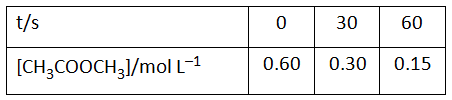# NEET Chemistry Chemical Kinetics Questions Solved

For the hydrolysis of methyl acetate in aqueous solution, the following results were obtained :(i) Show that it follows pseudo first order reaction, as the concentration of water remains constant.

(ii) Calculate the average rate of reaction between the time interval 30 to 60 seconds. (Given log 2 =

0.3010, log 4 = 0.6021)                         (5 marks)